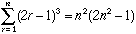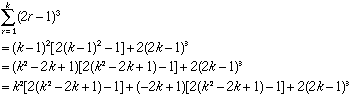Hi My name is Mr William, I am a student The question is secondary I am trying to prove a induction question Sigam r=1 n (2r -1)cube = n square (2 n square - 1) Thanks. Hi William, The expression you haveis certainly true when n = 1. Suppose now that the expression is true for n = k - 1, then for n = k,You should now be able to complete the problem. Cheers, Harley Go to Math Central